# HashMap 源码详细分析(JDK1.8)

## 原理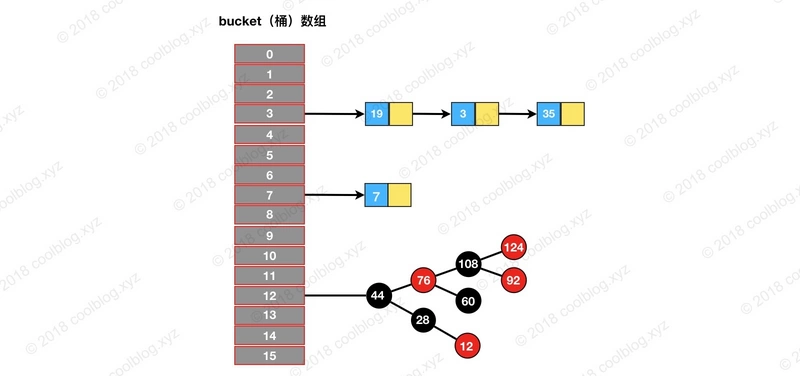• 定位元素35所处桶的位置，index = 35 % 16 = 3
• 在3号桶所指向的链表中继续查找，发现35在链表中。

## 源码分析

### 构造方法

#### 构造方法分析

HashMap 的构造方法不多，只有四个。HashMap 构造方法做的事情比较简单，一般都是初始化一些重要变量，比如 loadFactor 和 threshold。而底层的数据结构则是延迟到插入键值对时再进行初始化。HashMap 相关构造方法如下：

/** 构造方法 1 */
public HashMap() {
}

/** 构造方法 2 */
public HashMap(int initialCapacity) {
}

/** 构造方法 3 */
public HashMap(int initialCapacity, float loadFactor) {
if (initialCapacity < 0)
throw new IllegalArgumentException("Illegal initial capacity: " +
initialCapacity);
if (initialCapacity > MAXIMUM_CAPACITY)
initialCapacity = MAXIMUM_CAPACITY;
throw new IllegalArgumentException("Illegal load factor: " +
this.threshold = tableSizeFor(initialCapacity);
}

/** 构造方法 4 */
public HashMap(Map<? extends K, ? extends V> m) {
putMapEntries(m, false);
}


#### 初始容量、负载因子、阈值

initialCapacity HashMap 初始容量
threshold 当前 HashMap 所能容纳键值对数量的最大值，超过这个值，则需扩容

/** The default initial capacity - MUST be a power of two. */
static final int DEFAULT_INITIAL_CAPACITY = 1 << 4;

/** The load factor used when none specified in constructor. */
static final float DEFAULT_LOAD_FACTOR = 0.75f;

/** The next size value at which to resize (capacity * load factor). */
int threshold;


/**
* Returns a power of two size for the given target capacity.
*/
static final int tableSizeFor(int cap) {
int n = cap - 1;
n |= n >>> 1;
n |= n >>> 2;
n |= n >>> 4;
n |= n >>> 8;
n |= n >>> 16;
return (n < 0) ? 1 : (n >= MAXIMUM_CAPACITY) ? MAXIMUM_CAPACITY : n + 1;
}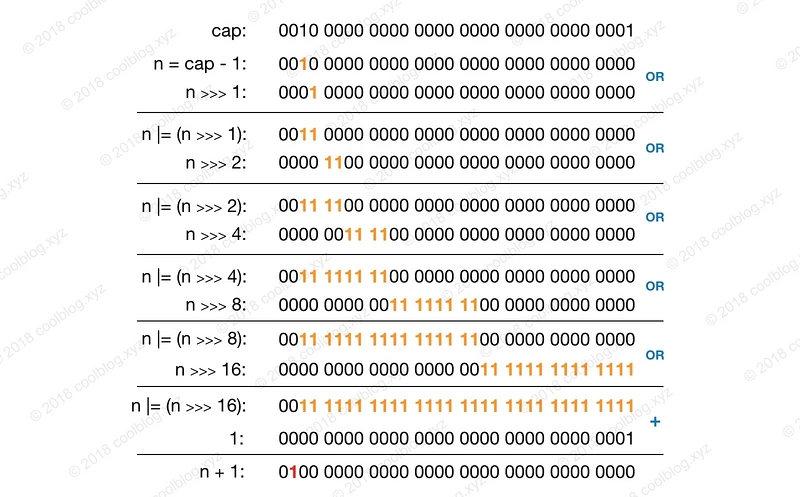## 查找

HashMap 的查找操作比较简单，查找步骤与原理篇介绍一致，即先定位键值对所在的桶的位置，然后再对链表或红黑树进行查找。通过这两步即可完成查找，该操作相关代码如下：

public V get(Object key) {
Node<K,V> e;
return (e = getNode(hash(key), key)) == null ? null : e.value;
}

final Node<K,V> getNode(int hash, Object key) {
Node<K,V>[] tab; Node<K,V> first, e; int n; K k;
// 1. 定位键值对所在桶的位置
if ((tab = table) != null && (n = tab.length) > 0 &&
(first = tab[(n - 1) & hash]) != null) {
if (first.hash == hash && // always check first node
((k = first.key) == key || (key != null && key.equals(k))))
return first;
if ((e = first.next) != null) {
// 2. 如果 first 是 TreeNode 类型，则调用黑红树查找方法
if (first instanceof TreeNode)
return ((TreeNode<K,V>)first).getTreeNode(hash, key);

// 2. 对链表进行查找
do {
if (e.hash == hash &&
((k = e.key) == key || (key != null && key.equals(k))))
return e;
} while ((e = e.next) != null);
}
}
return null;
}


// index = (n - 1) & hash
first = tab[(n - 1) & hash]/**
* 计算键的 hash 值
*/
static final int hash(Object key) {
int h;
return (key == null) ? 0 : (h = key.hashCode()) ^ (h >>> 16);
}## 遍历

for(Object key : map.keySet()) {
// do something
}


for(HashMap.Entry entry : map.entrySet()) {
// do something
}


Set keys = map.keySet();
Iterator ite = keys.iterator();
while (ite.hasNext()) {
Object key = ite.next();
// do something
}


public Set<K> keySet() {
Set<K> ks = keySet;
if (ks == null) {
ks = new KeySet();
keySet = ks;
}
return ks;
}

/**
* 键集合
*/
final class KeySet extends AbstractSet<K> {
public final int size()                 { return size; }
public final void clear()               { HashMap.this.clear(); }
public final Iterator<K> iterator()     { return new KeyIterator(); }
public final boolean contains(Object o) { return containsKey(o); }
public final boolean remove(Object key) {
return removeNode(hash(key), key, null, false, true) != null;
}
// 省略部分代码
}

/**
* 键迭代器
*/
final class KeyIterator extends HashIterator
implements Iterator<K> {
public final K next() { return nextNode().key; }
}

abstract class HashIterator {
Node<K,V> next;        // next entry to return
Node<K,V> current;     // current entry
int expectedModCount;  // for fast-fail
int index;             // current slot

HashIterator() {
expectedModCount = modCount;
Node<K,V>[] t = table;
current = next = null;
index = 0;
if (t != null && size > 0) { // advance to first entry
// 寻找第一个包含链表节点引用的桶
do {} while (index < t.length && (next = t[index++]) == null);
}
}

public final boolean hasNext() {
return next != null;
}

final Node<K,V> nextNode() {
Node<K,V>[] t;
Node<K,V> e = next;
if (modCount != expectedModCount)
throw new ConcurrentModificationException();
if (e == null)
throw new NoSuchElementException();
if ((next = (current = e).next) == null && (t = table) != null) {
// 寻找下一个包含链表节点引用的桶
do {} while (index < t.length && (next = t[index++]) == null);
}
return e;
}
//省略部分代码
}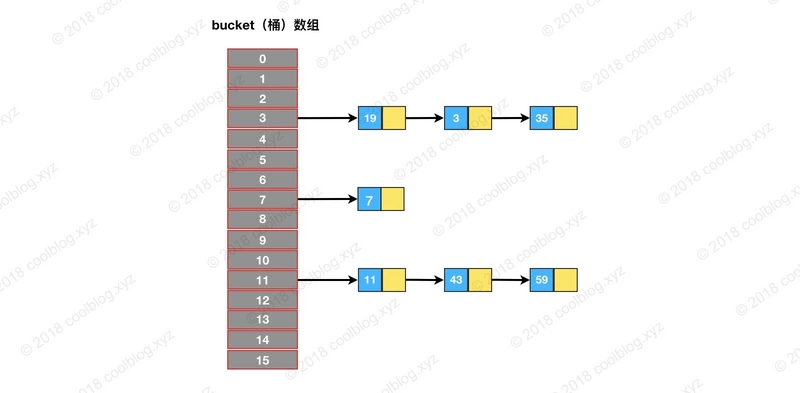HashIterator 在初始化时，会先遍历桶数组，找到包含链表节点引用的桶，对应图中就是3号桶。随后由 nextNode 方法遍历该桶所指向的链表。遍历完3号桶后，nextNode 方法继续寻找下一个不为空的桶，对应图中的7号桶。之后流程和上面类似，直至遍历完最后一个桶。以上就是 HashIterator 的核心逻辑的流程，对应下图：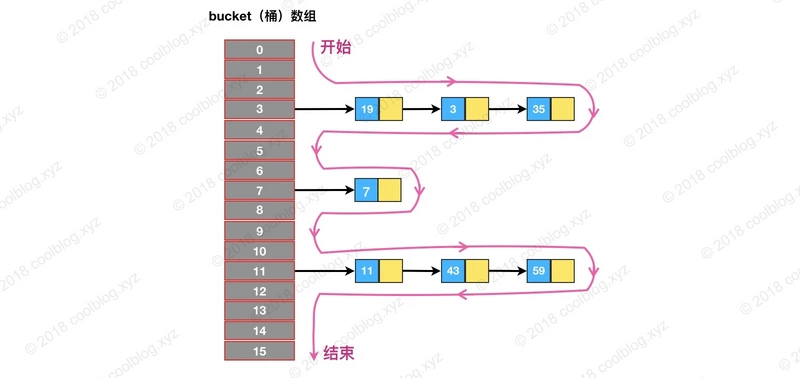/**
* 应在 JDK 1.8 下测试，其他环境下不保证结果和上面一致
*/
public class HashMapTest {

@Test
public void testTraversal() {
HashMap<Integer, String> map = new HashMap(16);
map.put(7, "");
map.put(11, "");
map.put(43, "");
map.put(59, "");
map.put(19, "");
map.put(3, "");
map.put(35, "");

System.out.println("遍历结果：");
for (Integer key : map.keySet()) {
System.out.print(key + " -> ");
}
}
}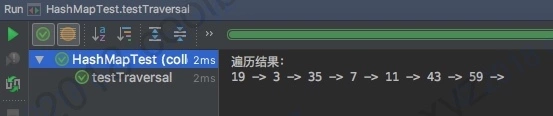## 插入

### 插入逻辑分析

public V put(K key, V value) {
return putVal(hash(key), key, value, false, true);
}

final V putVal(int hash, K key, V value, boolean onlyIfAbsent,
boolean evict) {
Node<K,V>[] tab; Node<K,V> p; int n, i;
// 初始化桶数组 table，table 被延迟到插入新数据时再进行初始化
if ((tab = table) == null || (n = tab.length) == 0)
n = (tab = resize()).length;
// 如果桶中不包含键值对节点引用，则将新键值对节点的引用存入桶中即可
if ((p = tab[i = (n - 1) & hash]) == null)
tab[i] = newNode(hash, key, value, null);
else {
Node<K,V> e; K k;
// 如果键的值以及节点 hash 等于链表中的第一个键值对节点时，则将 e 指向该键值对
if (p.hash == hash &&
((k = p.key) == key || (key != null && key.equals(k))))
e = p;

// 如果桶中的引用类型为 TreeNode，则调用红黑树的插入方法
else if (p instanceof TreeNode)
e = ((TreeNode<K,V>)p).putTreeVal(this, tab, hash, key, value);
else {
// 对链表进行遍历，并统计链表长度
for (int binCount = 0; ; ++binCount) {
// 链表中不包含要插入的键值对节点时，则将该节点接在链表的最后
if ((e = p.next) == null) {
p.next = newNode(hash, key, value, null);
// 如果链表长度大于或等于树化阈值，则进行树化操作
if (binCount >= TREEIFY_THRESHOLD - 1) // -1 for 1st
treeifyBin(tab, hash);
break;
}

// 条件为 true，表示当前链表包含要插入的键值对，终止遍历
if (e.hash == hash &&
((k = e.key) == key || (key != null && key.equals(k))))
break;
p = e;
}
}

// 判断要插入的键值对是否存在 HashMap 中
if (e != null) { // existing mapping for key
V oldValue = e.value;
// onlyIfAbsent 表示是否仅在 oldValue 为 null 的情况下更新键值对的值
if (!onlyIfAbsent || oldValue == null)
e.value = value;
afterNodeAccess(e);
return oldValue;
}
}
++modCount;
// 键值对数量超过阈值时，则进行扩容
if (++size > threshold)
resize();
afterNodeInsertion(evict);
return null;
}


• 1.当桶数组 table 为空时，通过扩容的方式初始化 table
• 2.查找要插入的键值对是否已经存在，存在的话根据条件判断是否用新值替换旧值
• 3.如果不存在，则将键值对链入链表中，并根据链表长度决定是否将链表转为红黑树
• 4.判断键值对数量是否大于阈值，大于的话则进行扩容操作

### 扩容机制

HashMap 的扩容机制与其他变长集合的套路不太一样，HashMap 按当前桶数组长度的2倍进行扩容，阈值也变为原来的2倍（如果计算过程中，阈值溢出归零，则按阈值公式重新计算）。扩容之后，要重新计算键值对的位置，并把它们移动到合适的位置上去。以上就是 HashMap 的扩容大致过程，接下来我们来看看具体的实现：

final Node<K,V>[] resize() {
Node<K,V>[] oldTab = table;
int oldCap = (oldTab == null) ? 0 : oldTab.length;
int oldThr = threshold;
int newCap, newThr = 0;
// 如果 table 不为空，表明已经初始化过了
if (oldCap > 0) {
// 当 table 容量超过容量最大值，则不再扩容
if (oldCap >= MAXIMUM_CAPACITY) {
threshold = Integer.MAX_VALUE;
return oldTab;
}
// 按旧容量和阈值的2倍计算新容量和阈值的大小
else if ((newCap = oldCap << 1) < MAXIMUM_CAPACITY &&
oldCap >= DEFAULT_INITIAL_CAPACITY)
newThr = oldThr << 1; // double threshold
} else if (oldThr > 0) // initial capacity was placed in threshold
/*
* 初始化时，将 threshold 的值赋值给 newCap，
* HashMap 使用 threshold 变量暂时保存 initialCapacity 参数的值
*/
newCap = oldThr;
else {               // zero initial threshold signifies using defaults
/*
* 调用无参构造方法时，桶数组容量为默认容量，
* 阈值为默认容量与默认负载因子乘积
*/
newCap = DEFAULT_INITIAL_CAPACITY;
}

// newThr 为 0 时，按阈值计算公式进行计算
if (newThr == 0) {
float ft = (float)newCap * loadFactor;
newThr = (newCap < MAXIMUM_CAPACITY && ft < (float)MAXIMUM_CAPACITY ?
(int)ft : Integer.MAX_VALUE);
}
threshold = newThr;
// 创建新的桶数组，桶数组的初始化也是在这里完成的
Node<K,V>[] newTab = (Node<K,V>[])new Node[newCap];
table = newTab;
if (oldTab != null) {
// 如果旧的桶数组不为空，则遍历桶数组，并将键值对映射到新的桶数组中
for (int j = 0; j < oldCap; ++j) {
Node<K,V> e;
if ((e = oldTab[j]) != null) {
oldTab[j] = null;
if (e.next == null)
newTab[e.hash & (newCap - 1)] = e;
else if (e instanceof TreeNode)
// 重新映射时，需要对红黑树进行拆分
((TreeNode<K,V>)e).split(this, newTab, j, oldCap);
else { // preserve order
Node<K,V> loHead = null, loTail = null;
Node<K,V> hiHead = null, hiTail = null;
Node<K,V> next;
// 遍历链表，并将链表节点按原顺序进行分组
do {
next = e.next;
if ((e.hash & oldCap) == 0) {
if (loTail == null)
else
loTail.next = e;
loTail = e;
}
else {
if (hiTail == null)
else
hiTail.next = e;
hiTail = e;
}
} while ((e = next) != null);
// 将分组后的链表映射到新桶中
if (loTail != null) {
loTail.next = null;
}
if (hiTail != null) {
hiTail.next = null;
}
}
}
}
}
return newTab;
}


• 1.计算新桶数组的容量 newCap 和新阈值 newThr
• 2.根据计算出的 newCap 创建新的桶数组，桶数组 table 也是在这里进行初始化的
• 3.将键值对节点重新映射到新的桶数组里。如果节点是 TreeNode 类型，则需要拆分红黑树。如果是普通节点，则节点按原顺序进行分组。

// 第一个条件分支
if ( oldCap > 0) {
// 嵌套条件分支
if (oldCap >= MAXIMUM_CAPACITY) {...}
else if ((newCap = oldCap << 1) < MAXIMUM_CAPACITY &&
oldCap >= DEFAULT_INITIAL_CAPACITY) {...}
}
else if (oldThr > 0) {...}
else {...}

// 第二个条件分支
if (newThr == 0) {...}


oldCap > 0 桶数组 table 已经被初始化
oldThr > 0 threshold > 0，且桶数组未被初始化 调用 HashMap(int) 和 HashMap(int, float) 构造方法时会产生这种情况，此种情况下 newCap = oldThr，newThr 在第二个条件分支中算出
oldCap == 0 && oldThr == 0 桶数组未被初始化，且 threshold 为 0 调用 HashMap() 构造方法会产生这种情况。

oldCap >= 230 桶数组容量大于或等于最大桶容量 230 这种情况下不再扩容
newCap < 230 && oldCap > 16 新桶数组容量小于最大值，且旧桶数组容量大于 16 该种情况下新阈值 newThr = oldThr << 1，移位可能会导致溢出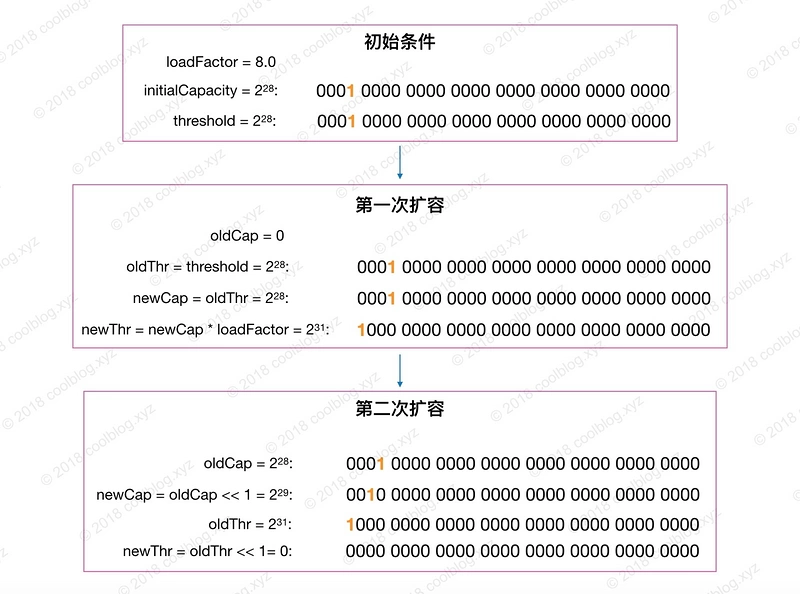newThr == 0 第一个条件分支未计算 newThr 或嵌套分支在计算过程中导致 newThr 溢出归零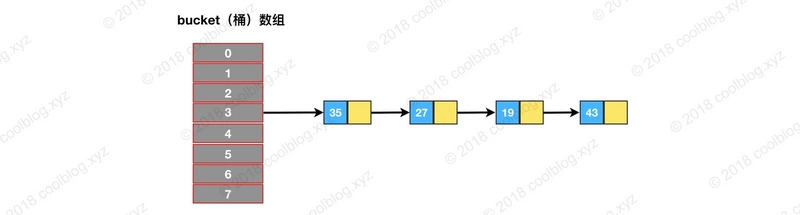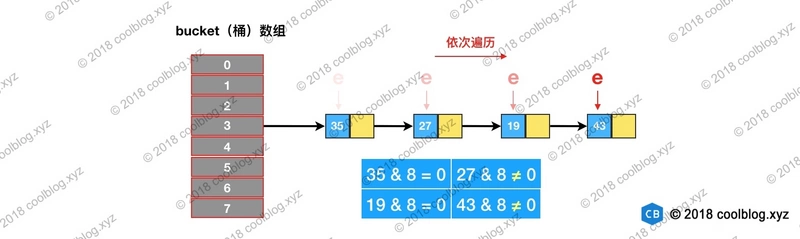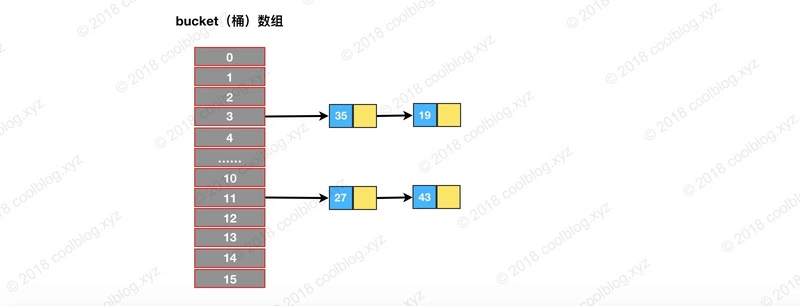### 链表树化、红黑树链化与拆分

JDK 1.8 对 HashMap 实现进行了改进。最大的改进莫过于在引入了红黑树处理频繁的碰撞，代码复杂度也随之上升。比如，以前只需实现一套针对链表操作的方法即可。而引入红黑树后，需要另外实现红黑树相关的操作。红黑树是一种自平衡的二叉查找树，本身就比较复杂。本篇文章中并不打算对红黑树展开介绍，本文仅会介绍链表树化需要注意的地方。至于红黑树详细的介绍，如果大家有兴趣，可以参考我的另一篇文章 - 红黑树详细分析。

static final int TREEIFY_THRESHOLD = 8;

/**
* 当桶数组容量小于该值时，优先进行扩容，而不是树化
*/
static final int MIN_TREEIFY_CAPACITY = 64;

static final class TreeNode<K,V> extends LinkedHashMap.Entry<K,V> {
TreeNode<K,V> parent;  // red-black tree links
TreeNode<K,V> left;
TreeNode<K,V> right;
TreeNode<K,V> prev;    // needed to unlink next upon deletion
boolean red;
TreeNode(int hash, K key, V val, Node<K,V> next) {
super(hash, key, val, next);
}
}

/**
* 将普通节点链表转换成树形节点链表
*/
final void treeifyBin(Node<K,V>[] tab, int hash) {
int n, index; Node<K,V> e;
// 桶数组容量小于 MIN_TREEIFY_CAPACITY，优先进行扩容而不是树化
if (tab == null || (n = tab.length) < MIN_TREEIFY_CAPACITY)
resize();
else if ((e = tab[index = (n - 1) & hash]) != null) {
TreeNode<K,V> hd = null, tl = null;
do {
// 将普通节点替换成树形节点
TreeNode<K,V> p = replacementTreeNode(e, null);
if (tl == null)
hd = p;
else {
p.prev = tl;
tl.next = p;
}
tl = p;
} while ((e = e.next) != null);  // 将普通链表转成由树形节点链表
if ((tab[index] = hd) != null)
// 将树形链表转换成红黑树
hd.treeify(tab);
}
}

TreeNode<K,V> replacementTreeNode(Node<K,V> p, Node<K,V> next) {
return new TreeNode<>(p.hash, p.key, p.value, next);
}


• 链表长度大于等于 TREEIFY_THRESHOLD
• 桶数组容量大于等于 MIN_TREEIFY_CAPACITY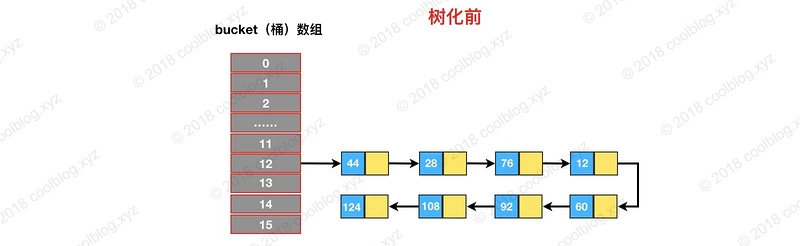HashMap 在设计之初，并没有考虑到以后会引入红黑树进行优化。所以并没有像 TreeMap 那样，要求键类实现 comparable 接口或提供相应的比较器。但由于树化过程需要比较两个键对象的大小，在键类没有实现 comparable 接口的情况下，怎么比较键与键之间的大小了就成了一个棘手的问题。为了解决这个问题，HashMap 是做了三步处理，确保可以比较出两个键的大小，如下：

• 比较键与键之间 hash 的大小，如果 hash 相同，继续往下比较
• 检测键类是否实现了 Comparable 接口，如果实现调用 compareTo 方法进行比较
• 如果仍未比较出大小，就需要进行仲裁了，仲裁方法为 tieBreakOrder（大家自己看源码吧）

tie break 是网球术语，可以理解为加时赛的意思，起这个名字还是挺有意思的。// 红黑树转链表阈值
static final int UNTREEIFY_THRESHOLD = 6;

final void split(HashMap<K,V> map, Node<K,V>[] tab, int index, int bit) {
TreeNode<K,V> b = this;
// Relink into lo and hi lists, preserving order
TreeNode<K,V> loHead = null, loTail = null;
TreeNode<K,V> hiHead = null, hiTail = null;
int lc = 0, hc = 0;
/*
* 红黑树节点仍然保留了 next 引用，故仍可以按链表方式遍历红黑树。
* 下面的循环是对红黑树节点进行分组，与上面类似
*/
for (TreeNode<K,V> e = b, next; e != null; e = next) {
next = (TreeNode<K,V>)e.next;
e.next = null;
if ((e.hash & bit) == 0) {
if ((e.prev = loTail) == null)
else
loTail.next = e;
loTail = e;
++lc;
}
else {
if ((e.prev = hiTail) == null)
else
hiTail.next = e;
hiTail = e;
++hc;
}
}

if (lc <= UNTREEIFY_THRESHOLD)
else {
/*
* 所有节点仍在原位置，树结构不变，无需重新树化
*/
}
}
// 与上面类似
if (hc <= UNTREEIFY_THRESHOLD)
else {
}
}
}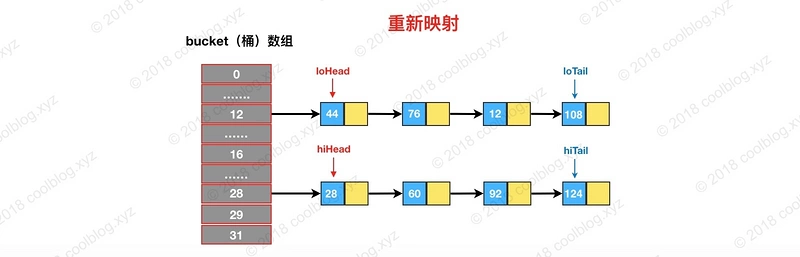final Node<K,V> untreeify(HashMap<K,V> map) {
Node<K,V> hd = null, tl = null;
// 遍历 TreeNode 链表，并用 Node 替换
for (Node<K,V> q = this; q != null; q = q.next) {
// 替换节点类型
Node<K,V> p = map.replacementNode(q, null);
if (tl == null)
hd = p;
else
tl.next = p;
tl = p;
}
return hd;
}

Node<K,V> replacementNode(Node<K,V> p, Node<K,V> next) {
return new Node<>(p.hash, p.key, p.value, next);
}


## 删除

HashMap 的删除操作并不复杂，仅需三个步骤即可完成。第一步是定位桶位置，第二步遍历链表并找到键值相等的节点，第三步删除节点。相关源码如下：

public V remove(Object key) {
Node<K,V> e;
return (e = removeNode(hash(key), key, null, false, true)) == null ?
null : e.value;
}

final Node<K,V> removeNode(int hash, Object key, Object value,
boolean matchValue, boolean movable) {
Node<K,V>[] tab; Node<K,V> p; int n, index;
if ((tab = table) != null && (n = tab.length) > 0 &&
// 1. 定位桶位置
(p = tab[index = (n - 1) & hash]) != null) {
Node<K,V> node = null, e; K k; V v;
// 如果键的值与链表第一个节点相等，则将 node 指向该节点
if (p.hash == hash &&
((k = p.key) == key || (key != null && key.equals(k))))
node = p;
else if ((e = p.next) != null) {
// 如果是 TreeNode 类型，调用红黑树的查找逻辑定位待删除节点
if (p instanceof TreeNode)
node = ((TreeNode<K,V>)p).getTreeNode(hash, key);
else {
// 2. 遍历链表，找到待删除节点
do {
if (e.hash == hash &&
((k = e.key) == key ||
(key != null && key.equals(k)))) {
node = e;
break;
}
p = e;
} while ((e = e.next) != null);
}
}

// 3. 删除节点，并修复链表或红黑树
if (node != null && (!matchValue || (v = node.value) == value ||
(value != null && value.equals(v)))) {
if (node instanceof TreeNode)
((TreeNode<K,V>)node).removeTreeNode(this, tab, movable);
else if (node == p)
tab[index] = node.next;
else
p.next = node.next;
++modCount;
--size;
afterNodeRemoval(node);
return node;
}
}
return null;
}


## 其他细节

• 1.table 多数情况下是无法被存满的，序列化未使用的部分，浪费空间
• 2.同一个键值对在不同 JVM 下，所处的桶位置可能是不同的，在不同的 JVM 下反序列化 table 可能会发生错误。

## 写在最后

posted @ 2019-01-13 16:21  陈树义  阅读(1451)  评论(0编辑  收藏  举报